# 1.11. 集成方法

• 平均方法，该方法的原理是构建多个独立的估计器，然后取它们的预测结果的平均。一般来说组合之后的估计器是会比单个估计器要好的，因为它的方差减小了。

示例: Bagging 方法 , 随机森林 , …

• 相比之下，在 boosting 方法 中，基估计器是依次构建的，并且每一个基估计器都尝试去减少组合估计器的偏差。这种方法主要目的是为了结合多个弱模型，使集成的模型更加强大。

示例: AdaBoost , 梯度提升树 , …

## 1.11.1. Bagging meta-estimator（Bagging 元估计器）

bagging 方法有很多种，其主要区别在于随机抽取训练子集的方法不同：

• 如果抽取的数据集的随机子集是样例的随机子集，我们叫做粘贴 (Pasting) [B1999] 。
• 如果样例抽取是有放回的，我们称为 Bagging [B1996] 。
• 如果抽取的数据集的随机子集是特征的随机子集，我们叫做随机子空间 (Random Subspaces) [H1998] 。
• 最后，如果基估计器构建在对于样本和特征抽取的子集之上时，我们叫做随机补丁 (Random Patches) [LG2012]。

>>> from sklearn.ensemble import BaggingClassifier
>>> from sklearn.neighbors import KNeighborsClassifier
>>> bagging = BaggingClassifier(KNeighborsClassifier(),
...                             max_samples=0.5, max_features=0.5)



## 1.11.2. 由随机树组成的森林

sklearn.ensemble 模块包含两个基于 随机决策树 的平均算法： RandomForest 算法和 Extra-Trees 算法。 这两种算法都是专门为树而设计的扰动和组合技术（perturb-and-combine techniques） [B1998] 。 这种技术通过在分类器构造过程中引入随机性来创建一组不同的分类器。集成分类器的预测结果就是单个分类器预测结果的平均值。

>>> from sklearn.ensemble import RandomForestClassifier
>>> X = [[0, 0], [1, 1]]
>>> Y = [0, 1]
>>> clf = RandomForestClassifier(n_estimators=10)
>>> clf = clf.fit(X, Y)



### 1.11.2.2. 极限随机树

>>> from sklearn.model_selection import cross_val_score
>>> from sklearn.datasets import make_blobs
>>> from sklearn.ensemble import RandomForestClassifier
>>> from sklearn.ensemble import ExtraTreesClassifier
>>> from sklearn.tree import DecisionTreeClassifier

>>> X, y = make_blobs(n_samples=10000, n_features=10, centers=100,
...     random_state=0)

>>> clf = DecisionTreeClassifier(max_depth=None, min_samples_split=2,
...     random_state=0)
>>> scores = cross_val_score(clf, X, y, cv=5)
>>> scores.mean()
0.98...

>>> clf = RandomForestClassifier(n_estimators=10, max_depth=None,
...     min_samples_split=2, random_state=0)
>>> scores = cross_val_score(clf, X, y, cv=5)
>>> scores.mean()
0.999...

>>> clf = ExtraTreesClassifier(n_estimators=10, max_depth=None,
...     min_samples_split=2, random_state=0)
>>> scores = cross_val_score(clf, X, y, cv=5)
>>> scores.mean() > 0.999
True### 1.11.2.5. 特征重要性评估• [L2014] G. Louppe, “Understanding Random Forests: From Theory to Practice”, PhD Thesis, U. of Liege, 2014.

### 1.11.2.6. 完全随机树嵌入

RandomTreesEmbedding 实现了一个无监督的数据转换。 通过由完全随机树构成的森林，RandomTreesEmbedding 使用数据最终归属的叶子节点的索引值（编号）对数据进行编码。 该索引以 one-of-K 方式编码，最终形成一个高维的稀疏二进制编码。 这种编码可以被非常高效地计算出来，并且可以作为其他学习任务的基础。 编码的大小和稀疏度可以通过选择树的数量和每棵树的最大深度来确定。对于集成中的每棵树的，每个样本对应其中的一个叶节点。 编码的大小（维度）最多为 n_estimators * 2 ** max_depth ，即森林中的叶子节点的最大数。

AdaBoost 的核心思想是用反复修改的数据（校对者注：主要是修正数据的权重）来训练一系列的弱学习器(一个弱学习器模型仅仅比随机猜测好一点, 比如一个简单的决策树),由这些弱学习器的预测结果通过加权投票(或加权求和)的方式组合, 得到我们最终的预测结果。在每一次所谓的提升（boosting）迭代中，数据的修改由应用于每一个训练样本的（新） 的权重,, …,组成（校对者注：即修改每一个训练样本应用于新一轮学习器的权重）。 初始化时,将所有弱学习器的权重都设置为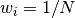,因此第一次迭代仅仅是通过原始数据训练出一个弱学习器。在接下来的 连续迭代中,样本的权重逐个地被修改,学习算法也因此要重新应用这些已经修改的权重。在给定的一个迭代中, 那些在上一轮迭代中被预测为错误结果的样本的权重将会被增加，而那些被预测为正确结果的样本的权 重将会被降低。随着迭代次数的增加，那些难以预测的样例的影响将会越来越大，每一个随后的弱学习器都将 会被强迫更加关注那些在之前被错误预测的样例 [HTF].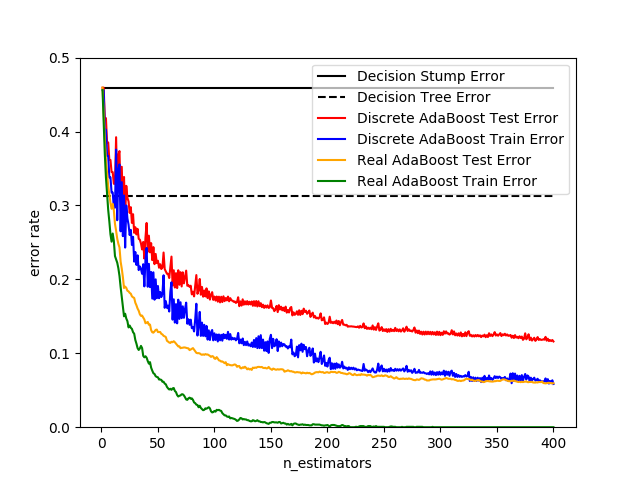### 1.11.3.1. 使用方法

>>> from sklearn.model_selection import cross_val_score

>>> scores = cross_val_score(clf, iris.data, iris.target)
>>> scores.mean()
0.9...



Gradient Tree Boosting 或梯度提升回归树（GBRT）是对于任意的可微损失函数的提升算法的泛化。 GBRT 是一个准确高效的现有程序， 它既能用于分类问题也可以用于回归问题。梯度树提升模型被应用到各种领域，包括网页搜索排名和生态领域。

GBRT 的优点:

• 对混合型数据的自然处理（异构特征）
• 强大的预测能力
• 在输出空间中对异常点的鲁棒性(通过具有鲁棒性的损失函数实现)

GBRT 的缺点:

• 可扩展性差（校对者注：此处的可扩展性特指在更大规模的数据集/复杂度更高的模型上使用的能力，而非我们通常说的功能的扩展性；GBRT 支持自定义的损失函数，从这个角度看它的扩展性还是很强的！）。由于提升算法的有序性(也就是说下一步的结果依赖于上一步)，因此很难做并行.

# explicitly require this experimental feature

from sklearn.experimental import enable_hist_gradient_boosting # noqa

# now you can import normally from ensemble

from sklearn.ensemble import HistGradientBoostingClassifier  下面的指南只关注GradientBoostingClassifierGradientBoostingRegressor，这可能是小样本量的首选，因为在这个设置中，装箱可能会导致分割点过于接近。

### 1.11.4.1. 分类

GradientBoostingClassifier 既支持二分类又支持多分类问题。 下面的例子展示了如何训练一个包含 100 个决策树弱学习器的梯度提升分类器:

>>> from sklearn.datasets import make_hastie_10_2

>>> X, y = make_hastie_10_2(random_state=0)
>>> X_train, X_test = X[:2000], X[2000:]
>>> y_train, y_test = y[:2000], y[2000:]

...     max_depth=1, random_state=0).fit(X_train, y_train)
>>> clf.score(X_test, y_test)
0.913...



### 1.11.4.2. 回归

>>> import numpy as np
>>> from sklearn.metrics import mean_squared_error
>>> from sklearn.datasets import make_friedman1

>>> X, y = make_friedman1(n_samples=1200, random_state=0, noise=1.0)
>>> X_train, X_test = X[:200], X[200:]
>>> y_train, y_test = y[:200], y[200:]
...     max_depth=1, random_state=0, loss='ls').fit(X_train, y_train)
>>> mean_squared_error(y_test, est.predict(X_test))
5.00...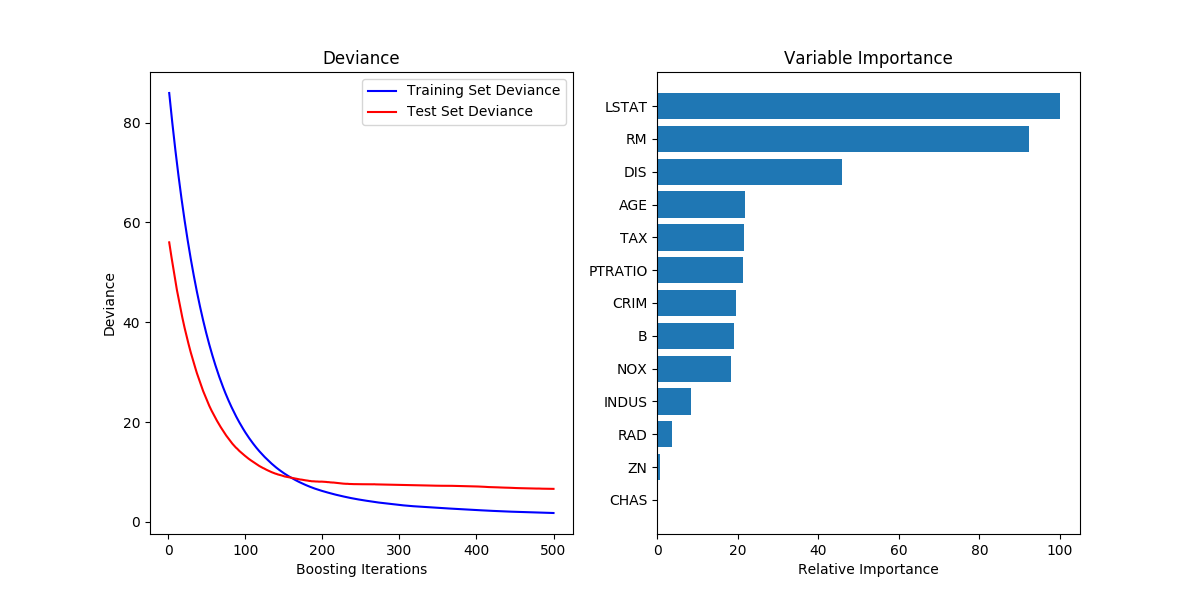### 1.11.4.3. 训练额外的弱学习器

GradientBoostingRegressorGradientBoostingClassifier 都支持设置参数 warm_start=True ，这样设置允许我们在已经训练的模型上面添加更多的估计器。

>>> _ = est.set_params(n_estimators=200, warm_start=True)  # set warm_start and new nr of trees
>>> _ = est.fit(X_train, y_train) # fit additional 100 trees to est
>>> mean_squared_error(y_test, est.predict(X_test))
3.84...



### 1.11.4.5. Mathematical formulation（数学公式）

GBRT 可以认为是以下形式的可加模型: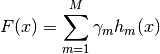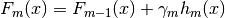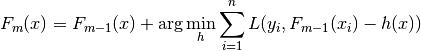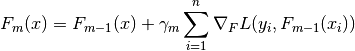#### 1.11.4.5.1. Loss Functions（损失函数）

• 回归 (Regression)
• Least squares ( 'ls' ): 由于其优越的计算性能,该损失函数成为回归算法中的自然选择。 初始模型 （校对者注：即损失函数的初始值，下同） 通过目标值的均值给出。
• Least absolute deviation ( 'lad' ): 回归中具有鲁棒性的损失函数,初始模型通过目 标值的中值给出。
• Huber ( 'huber' ): 回归中另一个具有鲁棒性的损失函数,它是最小二乘和最小绝对偏差两者的结合. 其利用 alpha 来控制模型对于异常点的敏感度(详细介绍请参考 [F2001]).
• Quantile ( 'quantile' ): 分位数回归损失函数.用 0 &lt; alpha &lt; 1 来指定分位数这个损 失函数可以用来产生预测间隔。（详见 Prediction Intervals for Gradient Boosting Regression ）。
• 分类 (Classification)
• Binomial deviance ('deviance'): 对于二分类问题(提供概率估计)即负的二项 log 似然损失函数。模型以 log 的比值比来初始化。
• Multinomial deviance ('deviance'): 对于多分类问题的负的多项log似然损失函数具有 n_classes 个互斥的类。提供概率估计。 初始模型由每个类的先验概率给出.在每一次迭代中 n_classes 回归树被构建,这使得 GBRT 在处理多类别数据集时相当低效。
• Exponential loss ('exponential'): 与 AdaBoostClassifier 具有相同的损失函数。与 'deviance' 相比，对被错误标记的样本的鲁棒性较差，仅用于在二分类问题。

### 1.11.4.6. Regularization（正则化）

#### 1.11.4.6.1. 收缩率 （Shrinkage）

[F2001]提出一个简单的正则化策略,通过一个因子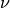来衡量每个弱分类器对于最终结果的贡献: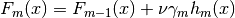#### 1.11.4.6.2. 子采样 （Subsampling）

[F1999]提出了随机梯度提升,这种方法将梯度提升（gradient boosting）和 bootstrap averaging(bagging) 相结合。在每次迭代中,基分类器是通过抽取所有可利用训练集中一小部分的 subsample 训练得到的子样本采用无放回的方式采样。 subsample 参数的值一般设置为 0.5 。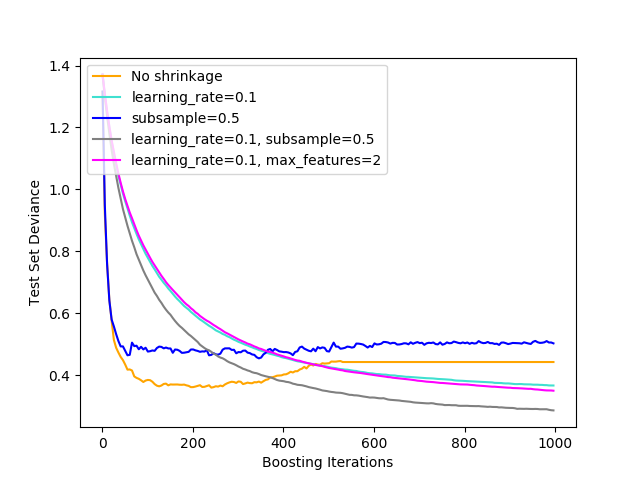### 1.11.4.7. Interpretation（解释性）

#### 1.11.4.7.1. Feature importance（特征重要性）

>>> from sklearn.datasets import make_hastie_10_2

>>> X, y = make_hastie_10_2(random_state=0)
...     max_depth=1, random_state=0).fit(X, y)
>>> clf.feature_importances_
array([0.10..., 0.10..., 0.11..., ...


## 1.11.5. Voting Classifier（投票分类器）

VotingClassifier （投票分类器）的原理是结合了多个不同的机器学习分类器,并且采用多数表决（majority vote）（硬投票） 或者平均预测概率（软投票）的方式来预测分类标签。 这样的分类器可以用于一组同样表现良好的模型,以便平衡它们各自的弱点。

### 1.11.5.1. 多数类标签 （又称为 多数/硬投票）

• classifier 1 -> class 1
• classifier 2 -> class 1
• classifier 3 -> class 2

• classifier 1 -> class 2
• classifier 2 -> class 1

#### 1.11.5.1.1. 用法

>>> from sklearn import datasets
>>> from sklearn.model_selection import cross_val_score
>>> from sklearn.linear_model import LogisticRegression
>>> from sklearn.naive_bayes import GaussianNB
>>> from sklearn.ensemble import RandomForestClassifier
>>> from sklearn.ensemble import VotingClassifier

>>> X, y = iris.data[:, 1:3], iris.target

>>> clf1 = LogisticRegression(solver='lbfgs', multi_class='multinomial',
...                           random_state=1)
>>> clf2 = RandomForestClassifier(n_estimators=50, random_state=1)
>>> clf3 = GaussianNB()

>>> eclf = VotingClassifier(estimators=[('lr', clf1), ('rf', clf2), ('gnb', clf3)], voting='hard')

>>> for clf, label in zip([clf1, clf2, clf3, eclf], ['Logistic Regression', 'Random Forest', 'naive Bayes', 'Ensemble']):
...     scores = cross_val_score(clf, X, y, cv=5, scoring='accuracy')
...     print("Accuracy: %0.2f (+/- %0.2f) [%s]" % (scores.mean(), scores.std(), label))
Accuracy: 0.95 (+/- 0.04) [Logistic Regression]
Accuracy: 0.94 (+/- 0.04) [Random Forest]
Accuracy: 0.91 (+/- 0.04) [naive Bayes]
Accuracy: 0.95 (+/- 0.04) [Ensemble]


### 1.11.5.2. 加权平均概率 （软投票）

>>> from sklearn import datasets
>>> from sklearn.tree import DecisionTreeClassifier
>>> from sklearn.neighbors import KNeighborsClassifier
>>> from sklearn.svm import SVC
>>> from itertools import product
>>> from sklearn.ensemble import VotingClassifier

>>> X = iris.data[:, [0, 2]]
>>> y = iris.target

>>> # Training classifiers
>>> clf1 = DecisionTreeClassifier(max_depth=4)
>>> clf2 = KNeighborsClassifier(n_neighbors=7)
>>> clf3 = SVC(gamma='scale', kernel='rbf', probability=True)
>>> eclf = VotingClassifier(estimators=[('dt', clf1), ('knn', clf2), ('svc', clf3)],
...                         voting='soft', weights=[2, 1, 2])

>>> clf1 = clf1.fit(X, y)
>>> clf2 = clf2.fit(X, y)
>>> clf3 = clf3.fit(X, y)
>>> eclf = eclf.fit(X, y)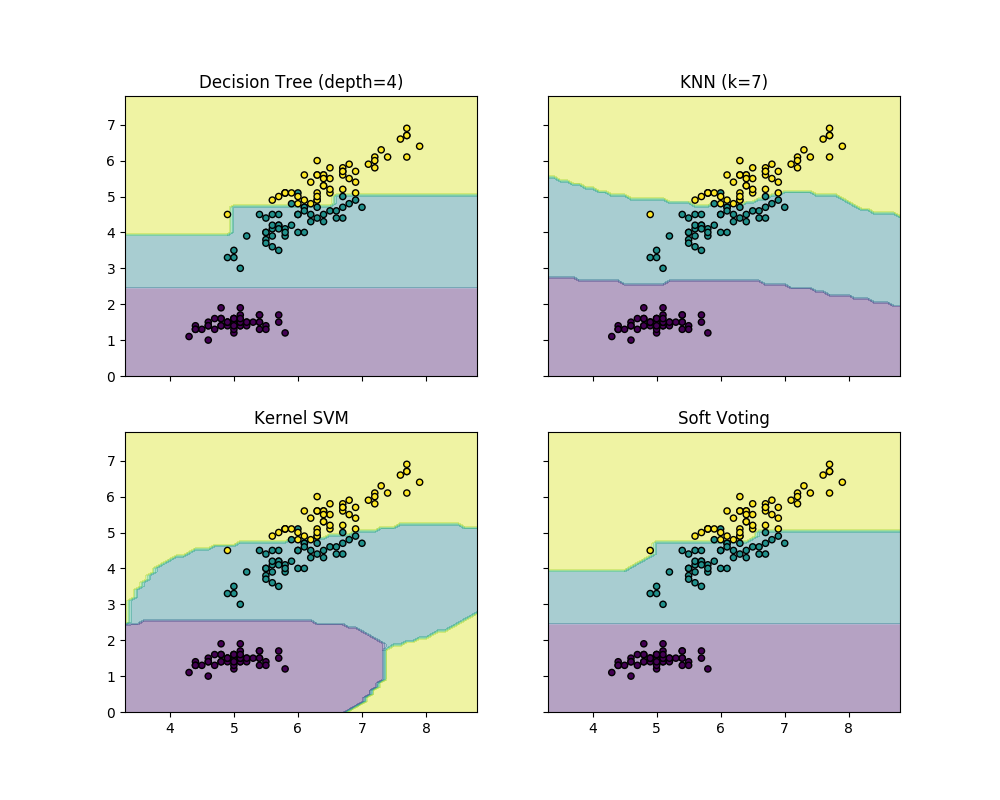### 1.11.5.3. 投票分类器（VotingClassifier）在网格搜索（GridSearchCV）应用

>>> from sklearn.model_selection import GridSearchCV
>>> clf1 = LogisticRegression(random_state=1)
>>> clf2 = RandomForestClassifier(random_state=1)
>>> clf3 = GaussianNB()
>>> eclf = VotingClassifier(estimators=[('lr', clf1), ('rf', clf2), ('gnb', clf3)], voting='soft')

>>> params = {'lr__C': [1.0, 100.0], 'rf__n_estimators': [20, 200],}

>>> grid = GridSearchCV(estimator=eclf, param_grid=params, cv=5)
>>> grid = grid.fit(iris.data, iris.target)



#### 1.11.5.3.1. 用法

>>> eclf = VotingClassifier(estimators=[('lr', clf1), ('rf', clf2), ('gnb', clf3)], voting='soft')



>>> eclf = VotingClassifier(estimators=[('lr', clf1), ('rf', clf2), ('gnb', clf3)], voting='soft', weights=[2,5,1])


## 1.11.6. 投票回归器(Voting Regressor)

>>> from sklearn import datasets
>>> from sklearn.ensemble import RandomForestRegressor
>>> from sklearn.linear_model import LinearRegression
>>> from sklearn.ensemble import VotingRegressor